JAVA语言之最基础的动态数据结构：链表### 什么是链表

```class Node {
E e;
Node next;  // 节点中持有下一个节点的引用}```• 优点：真正的动态结构，不需要处理固定容量的问题，从中间插入、删除节点很方便，相较于数组要灵活

• 缺点：丧失了随机访问的能力，不能像数组那种直接通过索引访问

```/**
* @program: Data-Structure
* @description: 链表数据结构实现
* @author: 01
* @create: 2018-11-08 15:37
* 链表中的节点结构
*/    private class Node {
E e;
Node next;        public Node() {            this(null, null);
}        public Node(E e) {            this(e, null);
}        public Node(E e, Node next) {            this.e = e;            this.next = next;
}        @Override        public String toString() {            return e.toString();
}
}    /**
* 头节点
* 链表中元素的个数
*/    private int size;    public LinkedList() {        this.head = null;        this.size = 0;
}    /**
* 获取链表中的元素个数
*
* @return 元素个数
*/    public int getSize() {        return size;
}    /**
* 链表是否为空
*
* @return 为空返回true，否则返回false
*/    public boolean isEmpty() {        return size == 0;
}
}```

### 在链表中添加元素```/**
* 在链表头添加新的元素e
*
* @param e 新的元素
Node node = new Node(e);

size++;
}``````/**
* 在链表的index(0-based)位置添加新的元素e
*
* @param index 元素添加的位置
* @param e     新的元素
*/public void add(int index, E e) {    // 检查索引是否合法    if (index < 0 || index > size) {        throw new IllegalArgumentException(""Add failed. Illegal index."");
}    // 链表头添加需特殊处理    if (index == 0) {
} else {
Node prev = head;        // 移动prev到index - 1的位置        for (int i = 0; i < index - 1; i++) {
prev = prev.next;
}

Node node = new Node(e);
node.next = prev.next;
prev.next = node;        // 同样，以上三句代码可以一句代码完成        // prev.next = new Node(e, prev.next);

size++;
}
}```

```/**
* 在链表末尾添加新的元素e
*
* @param e 新的元素
}```

### 使用链表的虚拟头节点```public class LinkedList<E> {
...    /**
* 虚拟头节点
* 链表中元素的个数
*/    private int size;    public LinkedList() {        this.dummyHead = new Node(null, null);        this.size = 0;
}    /**
* 在链表的index(0-based)位置添加新的元素e
*
* @param index 元素添加的位置
* @param e     新的元素
*/    public void add(int index, E e) {        if (index < 0 || index > size) {            throw new IllegalArgumentException(""Add failed. Illegal index."");
}

Node prev = dummyHead;        // 移动prev到index前一个节点的位置        for (int i = 0; i < index; i++) {
prev = prev.next;
}

Node node = new Node(e);
node.next = prev.next;
prev.next = node;        // 同样，以上三句代码可以一句代码完成        // prev.next = new Node(e, prev.next);

size++;
}    /**
* 在链表头添加新的元素e
*
* @param e 新的元素
*/    public void addFirst(E e) {
}    /**
* 在链表末尾添加新的元素e
*
* @param e 新的元素
*/    public void addLast(E e) {
}
}```

### 链表的遍历、查询和修改

```/**
* 获取链表的第index(0-based)个位置的元素
*
* @param index
* @return
*/public E get(int index) {    if (index < 0 || index >= size) {        throw new IllegalArgumentException(""Get failed. Illegal index."");
}

Node cur = dummyHead.next;    for (int i = 0; i < index; i++) {
cur = cur.next;
}    return cur.e;
}/**
* 获取链表中的第一个元素
*
* @return
*/public E getFirst() {    return get(0);
}/**
* 获取链表中的最后一个元素
*
* @return
*/public E getLast() {    return get(size - 1);
}/**
* 修改链表的第index(0-based)个位置的元素为e
*
* @param index
* @param e
*/public void set(int index, E e) {    if (index < 0 || index >= size) {        throw new IllegalArgumentException(""Set failed. Illegal index."");
}

Node cur = dummyHead.next;    for (int i = 0; i < index; i++) {
cur = cur.next;
}

cur.e = e;
}/**
* 查找链表中是否包含元素e
*
* @param e
* @return
*/public boolean contain(E e) {
Node cur = dummyHead.next;    // 第一种遍历链表的方式    while (cur != null) {        if (cur.e.equals(e)) {            return true;
}
cur = cur.next;
}    return false;
}@Overridepublic String toString() {    if (isEmpty()) {        return ""[]"";
}

StringBuilder sb = new StringBuilder();
sb.append(""["");
Node cur = dummyHead.next;    // 第二种遍历链表的方式    for (int i = 0; i < size; i++) {
sb.append(cur.e).append("" -> "");
cur = cur.next;
}    // 第三种遍历链表的方式    // for (Node cur = dummyHead.next; cur != null; cur = cur.next) {    //     sb.append(cur.e).append("" -> "");    // }    return sb.append(""NULL]"").toString();
}```

### 从链表中删除元素```/**
* 从链表中删除第index(0-based)个位置的元素，并返回删除的元素
*
* @param index
* @return 被删除的节点所存储的元素
*/public E remove(int index) {    if (index < 0 || index >= size) {        throw new IllegalArgumentException(""remove failed. Illegal index."");
}

Node prev = dummyHead;    for (int i = 0; i < index; i++) {
prev = prev.next;
}

Node delNode = prev.next;    // 把引用改变一下就完成了删除
prev.next = delNode.next;
delNode.next = null;
size--;    return delNode.e;
}```

```/**
* 删除链表中第一个元素
*
* @return 被删除的元素
*/public E removeFirst() {    return remove(0);
}/**
* 删除链表中最后一个元素
*
* @return 被删除的元素
*/public E removeLast() {    return remove(size - 1);
}```

`addLast(e)         // O(n)addFirst(e)        // O(1)add(index, e)      // O(n)removeLast()       // O(n)removeFirst()      // O(1)remove(index)      // O(n)set(index, e)      // O(n)get(index)         // O(n)contain(e)         // O(n)`

### 使用链表实现栈

```/**
* @program: Data-Structure
* @description: 基于链表实现栈数据结构
* @author: 01
* @create: 2018-11-08 23:38
}    @Override    public int getSize() {        return linkedList.getSize();
}    @Override    public boolean isEmpty() {        return linkedList.isEmpty();
}    @Override    public void push(E e) {
}    @Override    public E pop() {        return linkedList.removeFirst();
}    @Override    public E peek() {        return linkedList.getFirst();
}    @Override    public String toString() {        if (isEmpty()) {            return ""[]"";
}

StringBuilder sb = new StringBuilder();
sb.append(""top ["");        for (int i = 0; i < getSize(); i++) {
sb.append(linkedList.get(i));            if (i != getSize() - 1) {
sb.append("", "");
}
}        return sb.append(""]"").toString();
}    // 测试    public static void main(String[] args) {
Stack<Integer> stack = new LinkedListStack<>();        for (int i = 0; i < 5; i++) {
stack.push(i);
System.out.println(stack);
}

stack.pop();
System.out.println(stack);
}
}```

### 带有尾指针的链表：使用链表实现队列

```/**
* @program: Data-Structure
* @description: 基于链表实现的队列数据结构
* @author: 01
* @create: 2018-11-09 17:00
**/public class LinkedListQueue<E> implements Queue<E> {    private class Node {
E e;
Node next;        public Node() {            this(null, null);
}        public Node(E e) {            this(e, null);
}        public Node(E e, Node next) {            this.e = e;            this.next = next;
}        @Override        public String toString() {            return e.toString();
}
}    /**
* 头节点
* 尾节点
*/    private Node tail;    /**
* 表示队列中的元素个数
*/    private int size;    @Override    public void enqueue(E e) {        if (tail == null) {            // 链表没有元素
tail = new Node(e);
} else {            // 链尾入队元素
tail.next = new Node(e);
tail = tail.next;
}
size++;
}    @Override    public E dequeue() {        if (isEmpty()) {            throw new IllegalArgumentException(""Can't dequeue from an empty queue."");
}        // 链首出队元素
retNode.next = null;        if (head == null) {            // 队列里没元素的话，尾节点需要置空
tail = null;
}
size--;        return retNode.e;
}    @Override    public E getFront() {        if (isEmpty()) {            throw new IllegalArgumentException(""Queue is empty."");
}    @Override    public int getSize() {        return size;
}    @Override    public boolean isEmpty() {        return size == 0;
}    @Override    public String toString() {        if (isEmpty()) {            return ""[]"";
}

StringBuilder sb = new StringBuilder();
sb.append(""front ["");
Node cur = head;        while (cur != null) {
sb.append(cur.e).append("", "");
cur = cur.next;
}        return sb.append(""NULL] tail"").toString();
}
}```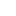喜欢 | 0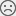不喜欢 | 0

2年研发与教学经验

• 10
文章
• 1576
人气
• 100%
受欢迎度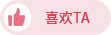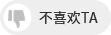•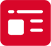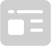索取资料
•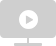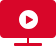答疑解惑
•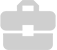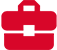技术交流
•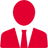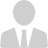职业测评
•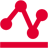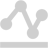面试技巧
•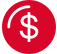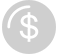高薪秘笈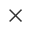Java全栈开发
WEB前端+H5沪公网安备 31011502005948号    ICP许可  沪B2-20190160